page 1:   1+1=2   1+2=3   1+3=4   1+4=5  1+5=6   1+6=7
page 2:   1+7=8   1+8=9   1+9=10   2+2=4   2+3=5   2+4=6
page 3:   2+5=7   2+6=8   2+7=9   2+8=10   3+3=6   3+4=7
page 4:   3+5=8   3+6=9   3+7=10   4+4=8   4+5=9   4+6=10
page 5:   5+5=10   2+9=11   3+8=11   4+7=11   5+6=11   3+9=12
page 6:   4+8=12   5+7=12   6+6=12   4+9=13   5+8=13   6+7=13
page 7:   5+9=14   6+8=14   7+7=14   6+9=15   7+8=15   7+9=16
page 8:   8+8=16   8+9=17   9+9=18
page 9:   6 blank cards
Printing and decorating the fact family cards:

* It is best to print the cards on thick paper such as card stock (used for report covers).
* Print the pages you need... skip the rest!
* If you prefer, you can print out several pages of blank cards and write your own numbers on the cards.
* On each fact family card, the smallest number is at the top left. If you would also like cards which place the smallest number at the top right, you may flip each card over and reverse the two top numbers, or you may print and write on blank cards.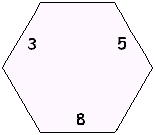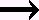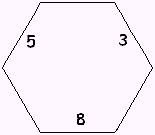* You might like to draw a picture of each fact on the front or the back of the card. Here are some ideas for illustrating one card: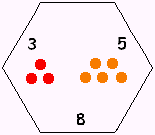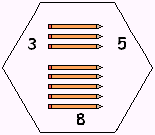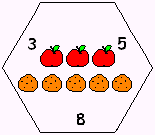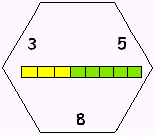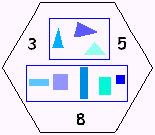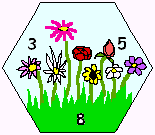Print out one or two fact family houses to hold the cards and hide one or two numbers at a time.

How to use the + - cards

multiplication / division cards